# MultiStep equations with fractions and decimals Solving OneStep

• Slides: 23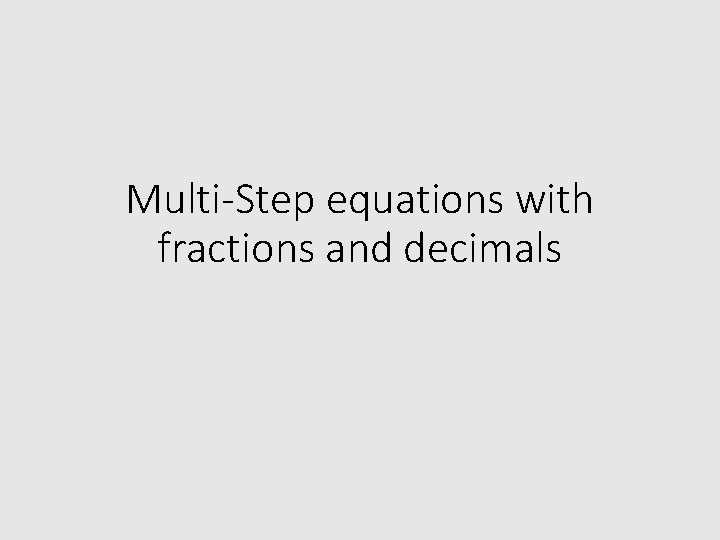Multi-Step equations with fractions and decimals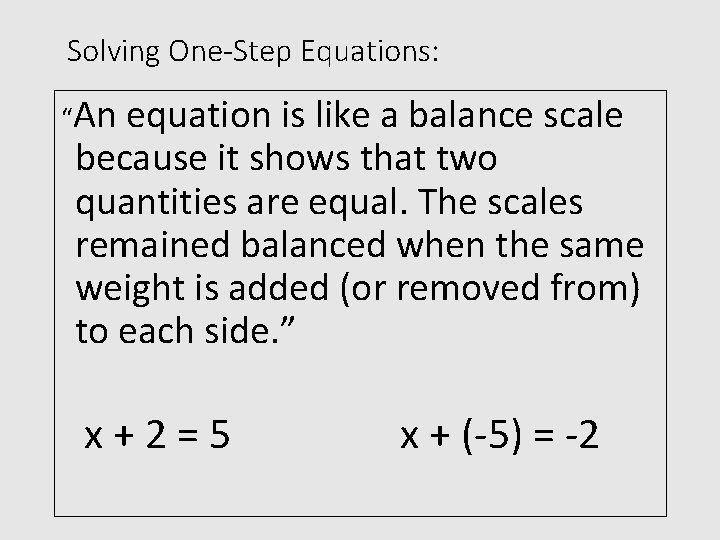Solving One-Step Equations: “An equation is like a balance scale because it shows that two quantities are equal. The scales remained balanced when the same weight is added (or removed from) to each side. ” x+2=5 x + (-5) = -2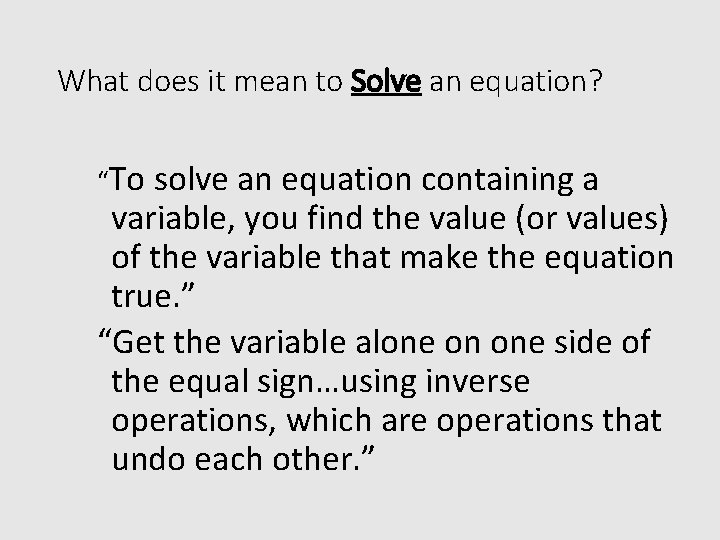What does it mean to Solve an equation? “To solve an equation containing a variable, you find the value (or values) of the variable that make the equation true. ” “Get the variable alone on one side of the equal sign…using inverse operations, which are operations that undo each other. ”Inverse Operations Addition and Subtraction are inverse operations because they undo each other. Multiplication and division are inverse operations because they undo each other.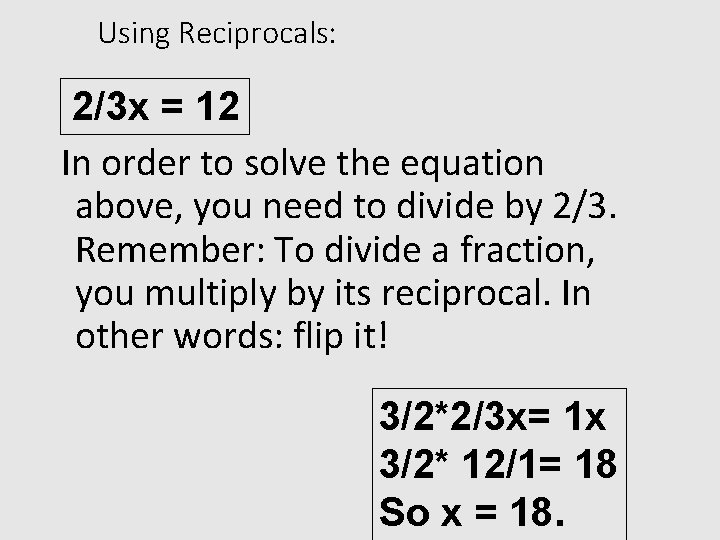Using Reciprocals: 2/3 x = 12 In order to solve the equation above, you need to divide by 2/3. Remember: To divide a fraction, you multiply by its reciprocal. In other words: flip it! 3/2*2/3 x= 1 x 3/2* 12/1= 18 So x = 18.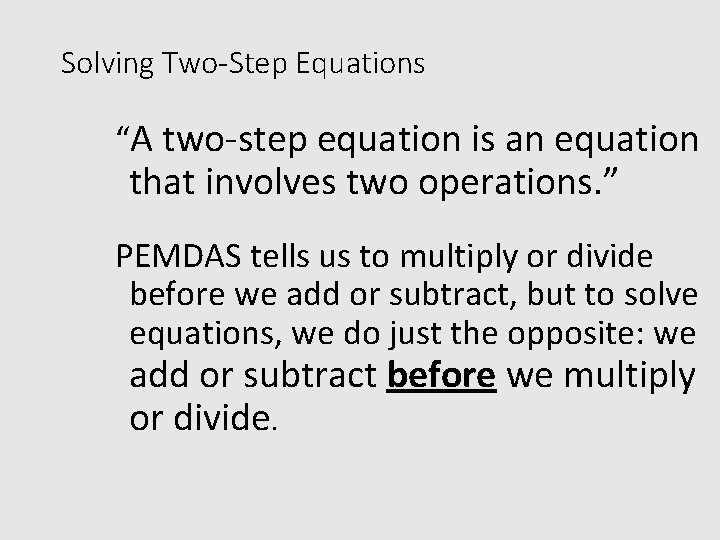Solving Two-Step Equations “A two-step equation is an equation that involves two operations. ” PEMDAS tells us to multiply or divide before we add or subtract, but to solve equations, we do just the opposite: we add or subtract before we multiply or divide.To Solve Multi-Step Equations: 1) “Clear the equation of fractions and decimals. ” 2) Apply the Distributive Property as needed. 3) “Combine like terms. ” 4) “Undo addition and subtraction. ” 5) “Undo multiplication and division. ”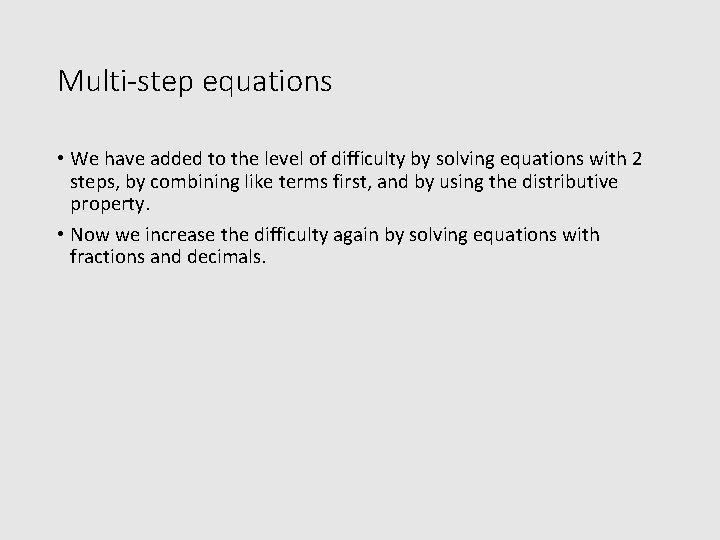Multi-step equations • We have added to the level of difficulty by solving equations with 2 steps, by combining like terms first, and by using the distributive property. • Now we increase the difficulty again by solving equations with fractions and decimals.Equations with Variables on Both Sides: Use the Addition or Subtraction property of Equality to get the variables on one side of the equation.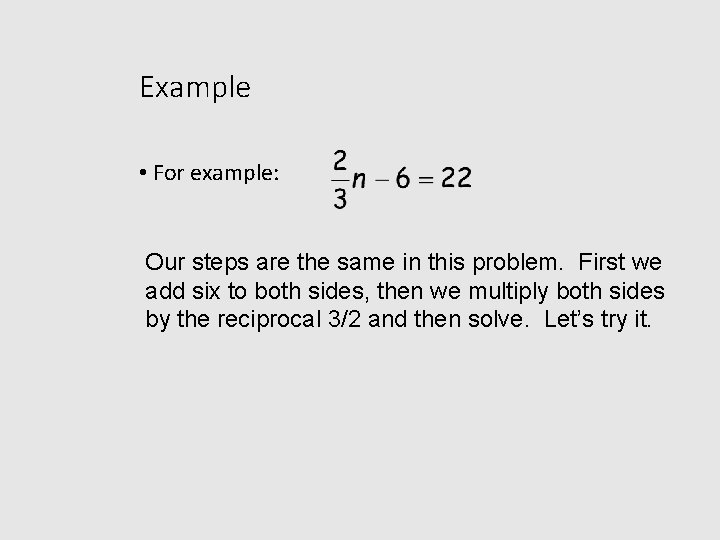Example • For example: Our steps are the same in this problem. First we add six to both sides, then we multiply both sides by the reciprocal 3/2 and then solve. Let’s try it.Let’s try +6 +6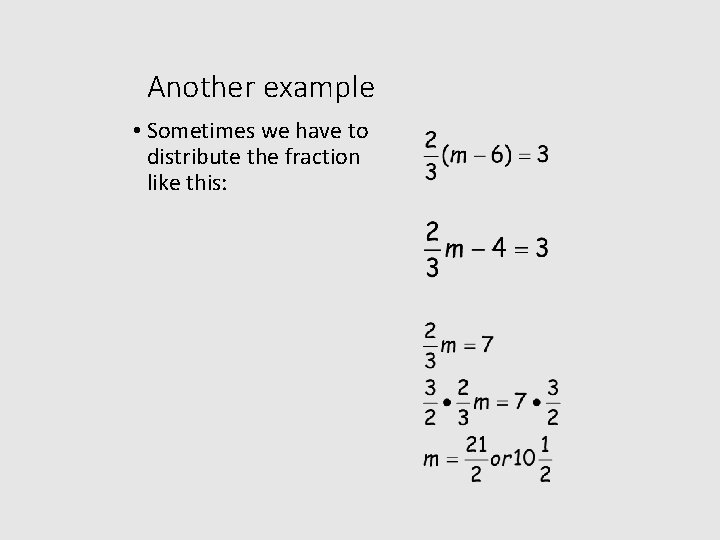Another example • Sometimes we have to distribute the fraction like this: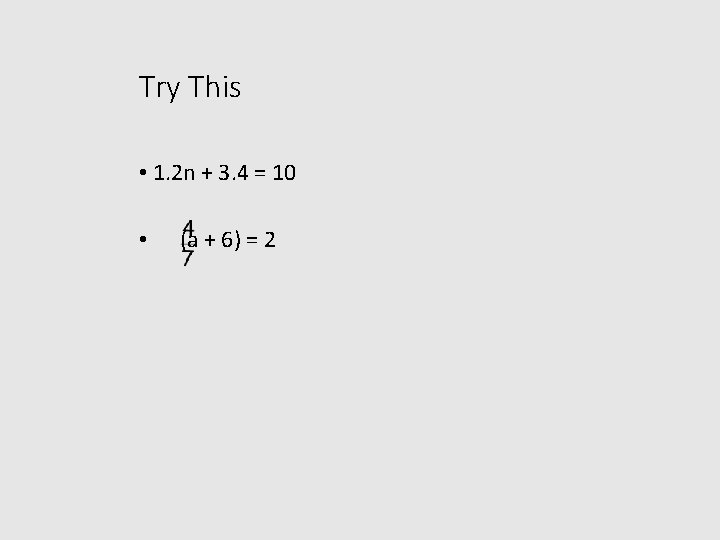Try This • 1. 2 n + 3. 4 = 10 • (a + 6) = 2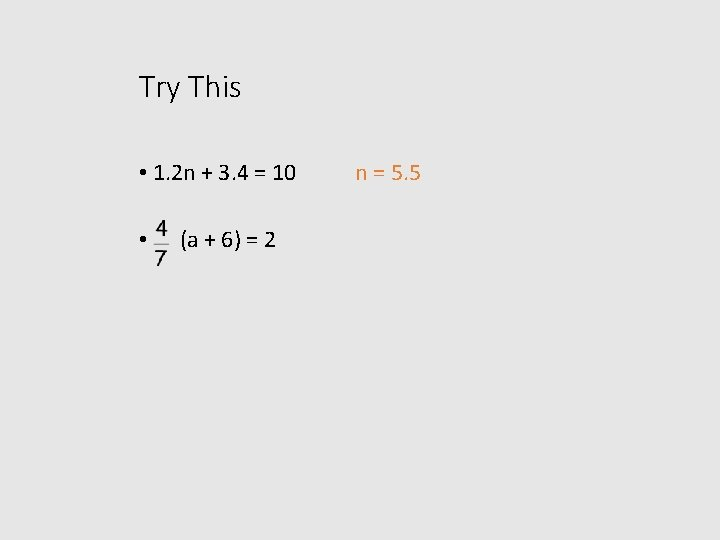Try This • 1. 2 n + 3. 4 = 10 • (a + 6) = 2 n = 5. 5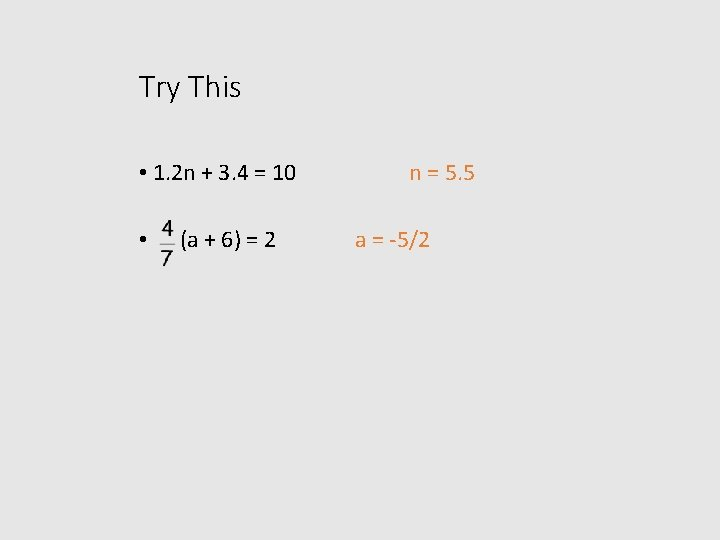Try This • 1. 2 n + 3. 4 = 10 • (a + 6) = 2 n = 5. 5 a = -5/2Another example First add 8 to both sides +8 +8 (2) Next multiply both sides by 2 Subtract 14 from both sides and solve. -14 N = 22Try This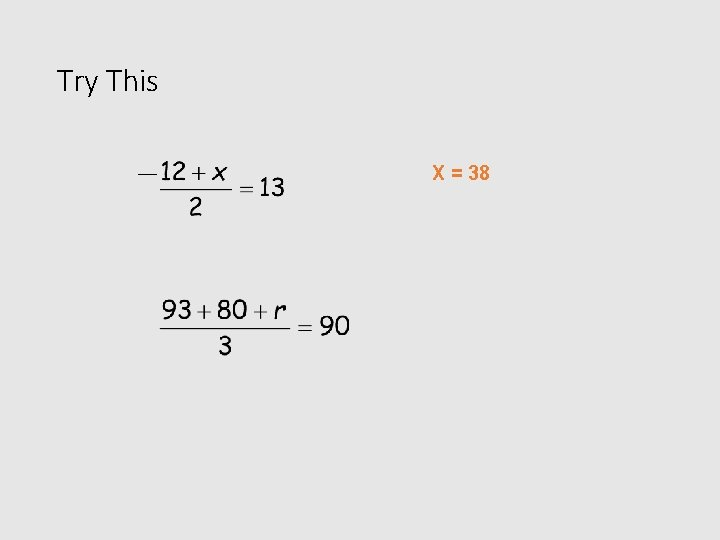Try This X = 38Try This X = -38 R = 97Clear the equation of fractions • Multiply each side by the LCD to get rid of the fraction or fractions.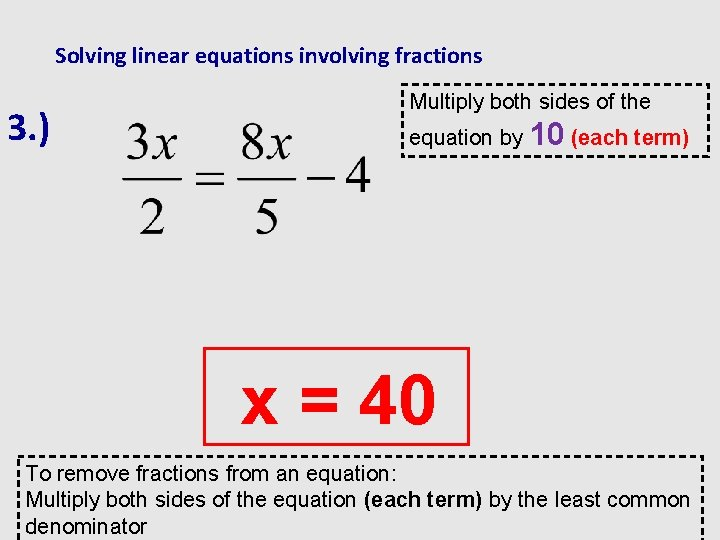Solving linear equations involving fractions 3. ) Multiply both sides of the equation by 10 (each term) x = 40 To remove fractions from an equation: Multiply both sides of the equation (each term) by the least common denominatorSolve 1. 2. 3. 4. 5. 6. 7. 8. 9. 10. Draw “the river” Clear the fraction – multiply each term by the LCD Simplify Add 2 x to both sides Simplify Add 6 to both sides Simplify Divide both sides by 6 Simplify Check your answer 3 - 2 x = 4 x – 6 + 2 x +2 x 3 = 6 x – 6 +6 +6 9 = 6 x 6 6 or 1. 5 = x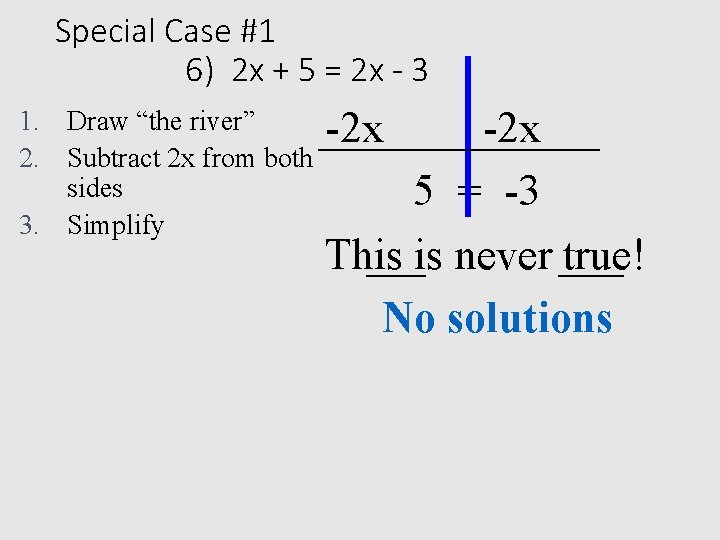Special Case #1 6) 2 x + 5 = 2 x - 3 1. Draw “the river” 2. Subtract 2 x from both sides 3. Simplify -2 x 5 = -3 This is never true! No solutions